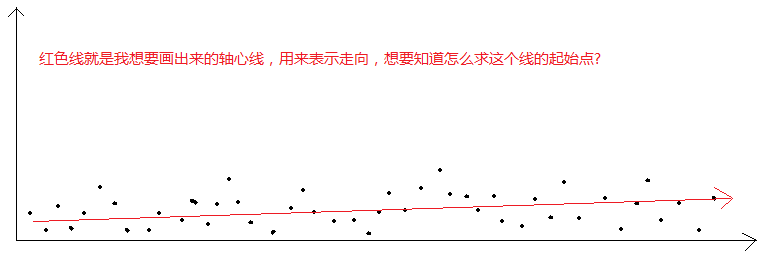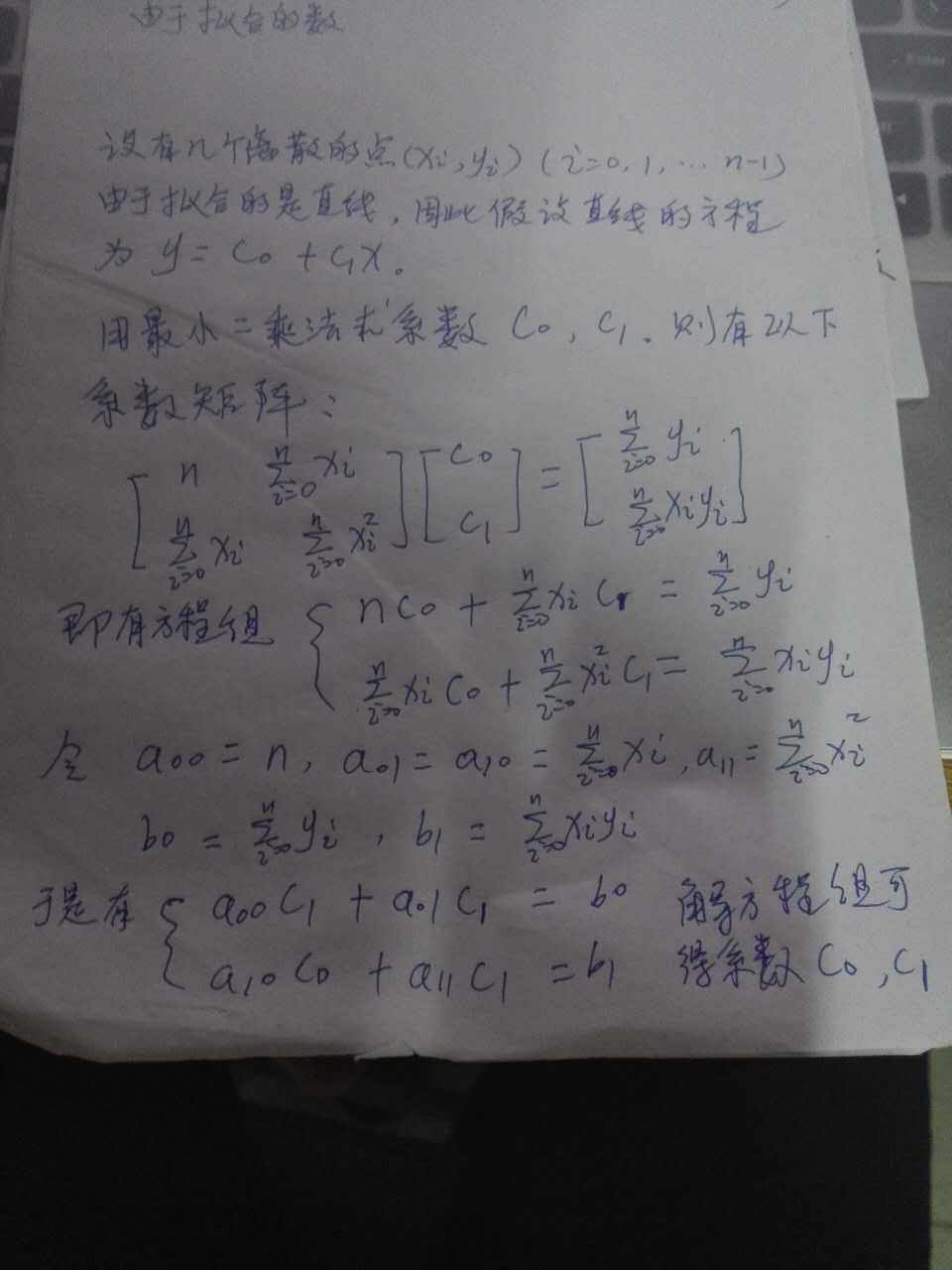2017-09-20 02:35

# 不限语言类型，想获得离散点集合的轴心线，以表示走向。• 点赞
• 写回答
• 关注问题
• 收藏
• 邀请回答

#### 3条回答默认 最新

•shifenglv 2017-09-20 03:26
已采纳

这是一个线性回归的问题。如果你只是想获得离散点的拟合曲线，用excel表格或者MATLAB就可以画出来，也可以得到拟合曲线的方程。如果你想知道生成拟合曲线的算法，你可以看看最小二乘法拟合曲线，如果是直线，算法还是很简单的。

点赞 打赏 评论
•椎名小由奈 2017-09-20 02:51

试试用c# 绘制来坐标

点赞 打赏 评论
•shifenglv 2017-09-20 13:31

最小二乘法拟合曲线的方法。我写的是求解拟合直线的系数的公式，未必能解释的清清楚楚，如果有什么困惑，还请参考书籍《数值计算方法》或者《数值方法简明教程》或者其他关于数值计算的书。下面是一段C程序，Python我没用过。程序并不复杂，你自己也可改为Python版本的代码。

`````` #include<stdio.h>
void Line(int n,double X[], double Y[], double *c0, double *c1);
int main()
{
double c0, c1;
double X = { 1,2,4,6,8,10 },Y = {1.8,3.7,8.2,12.0,15.8,20.2};

Line(6,X, Y, &c0, &c1);
printf("%lf %lf\n",c0,c1);
return 0;
}

//最小二乘法求拟合曲线
//输入：离散点的个数n,X变量，Y变量
//输出：直线y=c0+c1*x的两个系数c0和c1
void Line(int n,double X[], double Y[], double *c0, double *c1)//
{

double a00=0, a01=0, a10=0, a11=0, b0=0, b1=0;

a00 = n;

for (int i = 0;i < n;i++)
{
a01 = a01 + X[i];
a11 = a11 + X[i] * X[i];
b0 = b0 + Y[i];
b1 = b1 + X[i]*Y[i];
}

a10 = a01;

*c1 = (a00*b1 - a10*b0) / (a00*a11 - a10*a01);
*c0 = (b0 - a01*(*c1)) / a00;
}
``````
点赞 打赏 评论